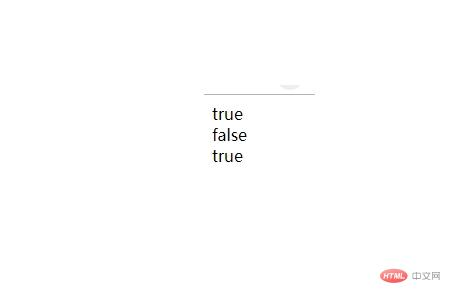# JavaScript 正则表达式前端控 更新时间：2019-02-14 11:55:05

JavaScript RegExp 对象

RegExp：是正则表达式（regular expression）的简写。

`var patt=new RegExp(pattern,modifiers);`

`var patt=/pattern/modifiers;`

`var re = new RegExp("\\w+");var re = /\w+/;`

RegExp 修饰符

i ： 修饰符是用来执行不区分大小写的匹配。

g ： 修饰符是用于执行全文的搜索（而不是在找到第一个就停止查找,而是找到所有的匹配）。

```<script>
var str = "hTmL中文网";
var patt1 = /html/i;
document.write(str.match(patt1));
</script>```

``` <script>
var str="Is this all there is?";
var patt1=/is/g;
document.write(str.match(patt1));
</script>```

```<script>
var str="Is this all there is?";
var patt1=/is/gi;
document.write(str.match(patt1));
</script>```

test()

test()方法搜索字符串指定的值，根据结果并返回真或假。

```<script>
var patt1=new RegExp("html");
document.write(patt1.test("html中文网"));
document.write(str.match(patt1));
</script>```

## 实例

```<script>
var str = 'runoob';
var patt1 = new RegExp('\\w', 'g'); // 有转义作为正则表达式处理
var patt2 = new RegExp('\w', 'g');  // 无转义作为字符串处理
var patt3 =/\w+/g;  // 与 patt1 效果相同
document.write(patt1.test(str)) //输出 true
document.write("<br>")
document.write(patt2.test(str)) //输出 false
document.write("<br>")
document.write(patt3.test(str)) //输出 true
</script>```exec()

exec() 方法检索字符串中的指定值。返回值是被找到的值。如果没有发现匹配，则返回 null。

```<script>
var patt1=new RegExp("m");
document.write(patt1.exec("html中文网"));
</script>```

php中文网
phpstudy

QQ交流群